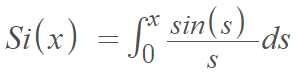# Sine Integral Function

The sine integral function, common in electrical engineering, is an odd function that sometimes arises when solving first order linear ordinary differential equations . It is defined by the integralThe integrand sin x ⁄ x is the sinc function.

In complex analysis, an alternative definition places infinity(∞) as the upper limit of the integral instead of x and also negates the integral :Both of the above definitions are analytics in the whole complex plane and are related by:## Derivative and Limits of the Sine Integral Function

The sine integral function is not defined when t = 0. However, the derivative isThis means that the limit as t → 0 = 1, so it’s usual to define f(0) = 1. This makes the function continuous everywhere (i.e., the set of all real numbers from -∞ to + ∞). .

## Local Maximums

Local maximums for the sine integral function are − 6π, − 4π, − 2π, π, 3π, 5π,… .

The sine integral function has local maximums where the derivative changes from positive to negative. To find these points, solve the first derivative for zero:The denominator cannot equal 0 (because division by zero is undefined), so we can simplify to 0 = sin(x). The derivative will change sign whenever sin(x) changes from positive to negative (giving us the places where sin(x) = 0).

1. x>0:. If x>0, the derivative’s denominator is positive. Sine functions are circular functions, based on the unit circle, so we know sin(x) will change from positive to negative at π, 3π, 5π,….
2. x<0: If x<0, the denominator is negative. Again, using the unit circle, these changes will happen at x = -2πr, -4π r, -6πr.

## Fresnel Sine Integral Function

The Fresnel sine integral function is given by :## References

 Sine Integral Function Si(x). Retrieved March 1, 2022 from: https://mattclay.hosted.uark.edu/Teaching/Spring2013/sineintegral.html
 Mathematical methods for wave propagation in science and engineering. Volume 1: Fundamentals. Eds. Universidad Católica de Chile.
 Calculus I, Section 5.3, #72. The Fundamental Theorem of Calculus. Retrieved March 1, 2022 from: https://sccollege.edu/Departments/MATH/Documents/Math%20180/05-03-072_The_Fundamental_Theorem_of_Calculus.pdf
 Attar, R. (2006). Special Functions and Orthogonal Polynomials. Lulu Press.

CITE THIS AS:
Stephanie Glen. "Sine Integral Function" From StatisticsHowTo.com: Elementary Statistics for the rest of us! https://www.statisticshowto.com/sine-integral-function/